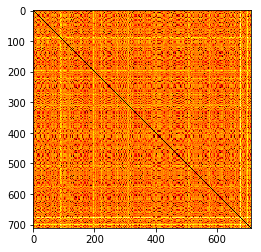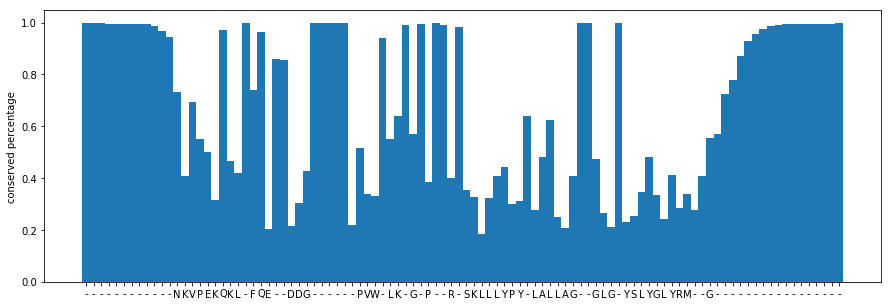# Test Installation

import sys
sys.version

'3.6.3 | packaged by conda-forge | (default, Nov  4 2017, 10:13:32) \n[GCC 4.2.1 Compatible Apple LLVM 6.1.0 (clang-602.0.53)]'

import numpy as np
np.version.version

'1.14.2'

import numba
numba.__version__

'0.36.2'

import math
import matplotlib.pyplot as plt
from collections import Counter

%matplotlib inline


# What is Numba

Numba gives you the power to speed up your applications with high performance functions written directly in Python. With a few annotations, array-oriented and math-heavy Python code can be just-in-time compiled to native machine instructions, similar in performance to C, C++ and Fortran, without having to switch languages or Python interpreters.

-Numba Website

## Numeric Data

### Pure Python

list_of_lists = [list(np.random.rand(2500)) for i in range(2500)]
print(len(list_of_lists), len(list_of_lists))

2500 2500

def python_sum_of_squares_list(input_arr):
result = 0
num_rows, num_cols = len(input_arr), len(input_arr)
for i in range(num_rows):
for j in range(num_cols):
result += input_arr[i][j]**2
return result

python_sum_of_squares_list(list_of_lists)

2082606.0782765413

%timeit -n 2 -r 2 python_sum_of_squares_list(list_of_lists)

2.64 s ± 24.4 ms per loop (mean ± std. dev. of 2 runs, 2 loops each)


### Using numpy arrays

numpy_array = np.array(list_of_lists)
print(numpy_array.shape)

(2500, 2500)

def numpy_loop_sum_of_squares_array(input_arr):
result = 0
num_rows, num_cols = input_arr.shape
for i in range(num_rows):
for j in range(num_cols):
result += input_arr[i, j]**2
return result

numpy_loop_sum_of_squares_array(numpy_array)

2082606.0782765413

%timeit -n 2 -r 2 numpy_loop_sum_of_squares_array(numpy_array)

3.45 s ± 65.3 ms per loop (mean ± std. dev. of 2 runs, 2 loops each)


### Numpy vectorization

def numpy_vectorized_sum_of_squares_array(input_arr):
return np.sum(input_arr**2)

numpy_vectorized_sum_of_squares_array(numpy_array)

2082606.0782765397

%timeit numpy_vectorized_sum_of_squares_array(numpy_array)

24 ms ± 654 µs per loop (mean ± std. dev. of 7 runs, 10 loops each)


### numba.jit

@numba.jit
def numba_sum_of_squares_array(input_arr):
result = 0
num_rows, num_cols = input_arr.shape
for i in range(num_rows):
for j in range(num_cols):
result += input_arr[i,j]**2
return result

numba_sum_of_squares_array(numpy_array)

2082606.0782765413

%timeit numba_sum_of_squares_array(numpy_array)

7.66 ms ± 341 µs per loop (mean ± std. dev. of 7 runs, 100 loops each)


### Contrived example:

2 matrices, if 1st > 2nd, 1st^2 - sqrt(2nd), otherwise 2nd^2 - sqrt(1st)

def square_or_sqrt(in1, in2):
num_rows, num_cols = in1.shape
output_arr = np.zeros((num_rows, num_cols))
for i in range(num_rows):
for j in range(num_cols):
if in1[i, j] > in2[i, j]:
output_arr[i, j] = (in1[i,j] ** 2) - math.sqrt(in2[i,j])
else:
output_arr[i, j] = (in2[i,j] ** 2) - math.sqrt(in1[i,j])
return output_arr

numpy_array_2 = np.random.rand(2500, 2500)

%timeit -n 1 -r 1 square_or_sqrt(numpy_array, numpy_array_2)

8.82 s ± 0 ns per loop (mean ± std. dev. of 1 run, 1 loop each)


### numba.vectorize

@numba.vectorize([numba.float64(numba.int64, numba.int64), numba.float64(numba.float64, numba.float64)])
def numba_square_or_sqrt(in1, in2):
if in1 > in2:
return (in1 ** 2) - math.sqrt(in2)
else:
return (in2 ** 2) - math.sqrt(in1)

%timeit numba_square_or_sqrt(numpy_array, numpy_array_2)

34.9 ms ± 4.5 ms per loop (mean ± std. dev. of 7 runs, 10 loops each)

p = square_or_sqrt(numpy_array, numpy_array_2)

n = numba_square_or_sqrt(numpy_array, numpy_array_2)

np.sum(p), np.sum(n)

(-207598.87433288957, -207598.87433288957)


## Sequence Data

sequence = np.random.choice(np.array(tuple("ACGT"), dtype="S1"), 50000000)
print(sequence[:21].view("S3"))

[b'GGT' b'GCC' b'GCC' b'AGC' b'AAC' b'TAG' b'AGG']

string_sequence = sequence.tostring().decode()
string_sequence[:20]

'GGTGCCGCCAGCAACTAGAG'


### Replace T with U

%timeit string_sequence.replace("T", "U")

184 ms ± 4.51 ms per loop (mean ± std. dev. of 7 runs, 10 loops each)

t = ord("T")
u = ord("U")
t, u

(84, 85)

@numba.vectorize([numba.int8(numba.int8)])
def replaceTU(x):
if x == t:
return u
else:
return x

%timeit replaceTU(sequence.view("int8"))

19.8 ms ± 474 µs per loop (mean ± std. dev. of 7 runs, 100 loops each)


### Kmerization

def python_kmerize(sequence, k):
length_sequence = len(sequence)
kmers = [sequence[i:i+k] for i in range(length_sequence-k+1)]
return kmers

%timeit python_kmerize(string_sequence, k=20)

20.9 s ± 1.04 s per loop (mean ± std. dev. of 7 runs, 1 loop each)

@numba.jit
def numba_kmerize(sequence, k):
kmers = np.zeros((sequence.shape-k+1, k), dtype="int8")
length_sequence = sequence.shape
for i in range(length_sequence-k+1):
kmers[i] = sequence[i: i+k]
return kmers

%timeit numba_kmerize(sequence.view("int8"), k=20)

2.45 s ± 60.9 ms per loop (mean ± std. dev. of 7 runs, 1 loop each)


### Parallel

parallel=True

numba.prange instead of range

numba.config.NUMBA_NUM_THREADS

4

@numba.jit(parallel=True)
def p_numba_kmerize(sequence, k):
kmers = np.zeros((sequence.shape-k+1, k), dtype="int8")
for i in numba.prange(sequence.shape-k+1):
kmers[i] = sequence[i: i+k]
return kmers

%timeit p_numba_kmerize(sequence.view("int8"), k=20)

781 ms ± 41.5 ms per loop (mean ± std. dev. of 7 runs, 1 loop each)


## Alignments

def get_sequences_from_fasta(fasta_file: str) -> dict:
sequences = {}
with open(fasta_file) as f:
current_sequence = ""
current_key = None
for line in f:
if line.startswith(">"):
if current_key is None:
current_key = line.split(">").strip()
else:
sequences[current_key] = current_sequence
current_sequence = ""
current_key = line.split(">").strip()
else:
current_sequence += line.strip()
sequences[current_key] = current_sequence
return sequences

sequences_fasta = get_sequences_from_fasta("PF02238_full.fasta")

keys = list(sequences_fasta.keys())
sequences = list(sequences_fasta.values())
n_sequences = np.array([[ord(x) for x in seq] for seq in sequences], dtype="int8")
n_sequences.shape

(711, 102)


#### Find pairwise distance matrix

def python_pairwise_distance(sequence_1, sequence_2):
num_mismatches = 0
length_sequence = len(sequence_1)
for i in range(length_sequence):
if sequence_1[i] != sequence_2[i]:
num_mismatches += 1
return num_mismatches/length_sequence

def python_distance_matrix(sequences):
num_sequences = len(sequences)
matrix = np.zeros((num_sequences, num_sequences))
for i in range(num_sequences):
for j in range(num_sequences):
matrix[i, j] = python_pairwise_distance(sequences[i], sequences[j])
return matrix

p = %timeit -o -n 2 -r 2 python_distance_matrix(sequences)

5.84 s ± 58.9 ms per loop (mean ± std. dev. of 2 runs, 2 loops each)

@numba.jit
def numba_pairwise_distance(sequence_1, sequence_2):
num_mismatches = 0
length_sequence = sequence_1.shape
for i in range(length_sequence):
if sequence_1[i] != sequence_2[i]:
num_mismatches += 1
return num_mismatches/length_sequence

@numba.jit(parallel=True)
def numba_distance_matrix(sequences):
num_sequences = sequences.shape
matrix = np.zeros((num_sequences, num_sequences))
for i in numba.prange(num_sequences):
for j in numba.prange(num_sequences):
matrix[i, j] = numba_pairwise_distance(sequences[i], sequences[j])
return matrix

n = %timeit -o numba_distance_matrix(n_sequences)

9.48 ms ± 212 µs per loop (mean ± std. dev. of 7 runs, 1 loop each)

p.best/n.best

626.3191265320067

n_distmat = numba_distance_matrix(n_sequences)

distmat = python_distance_matrix(sequences)

plt.imshow(n_distmat, cmap="hot")

<matplotlib.image.AxesImage at 0x109c55c18>plt.imshow(distmat, cmap="hot")

<matplotlib.image.AxesImage at 0x132d34828>#### Find consensus sequence and consensus amino acid frequencies

def python_consensus(sequences):
len_alignment = len(sequences)
num_sequences = len(sequences)
counter_per_position = []
for i in range(len_alignment):
counts = []
for j in range(num_sequences):
counts.append(sequences[j][i])
counter = Counter(counts)
for c in counter:
counter[c] /= len(counts)
counter_per_position.append(counter.most_common())
consensus_sequence = "".join(c for c in counter_per_position)
consensus_frequencies = [c for c in counter_per_position]
return consensus_sequence, consensus_frequencies

%timeit python_consensus(sequences)

17.6 ms ± 399 µs per loop (mean ± std. dev. of 7 runs, 100 loops each)

py_seq, py_freqs = python_consensus(sequences)

@numba.jit(parallel=True)
def numba_consensus(sequences):
sequences_t = sequences.T
consensus = np.zeros((sequences_t.shape, 256), dtype="i8")
for i in numba.prange(sequences_t.shape):
for letter in sequences_t[i]:
consensus[i,letter] += 1
return np.argmax(consensus, axis=1).astype("int8").view("S1"), np.max(consensus, axis=1)/sequences_t.shape

%timeit numba_consensus(n_sequences)

241 µs ± 37.9 µs per loop (mean ± std. dev. of 7 runs, 1 loop each)

n_seq, n_freqs = numba_consensus(n_sequences)
n_seq = n_seq.tostring().decode()

def plot_consensus(consensus_sequence, consensus_frequencies):
plt.bar(range(len(consensus_sequence)), consensus_frequencies, width=1)
plt.xticks(range(len(consensus_sequence)), list(consensus_sequence))
plt.ylabel("conserved percentage")

plt.figure(figsize=(15,5))
plot_consensus(n_seq[:100], n_freqs[:100])## Using more than one element

Use neighboring elements, kernels, by row, by column etc.

## Classes

@numba.jitclass

Let’s you use python class syntax (methods, properties/attributes)

## C functions

@numba.cfunc

• C-functions with pure Python syntax
• Cleaner syntax than Cython
• Can go low-level